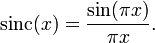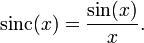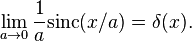## Thursday, November 18, 2010

### Sinc Function

http://en.wikipedia.org/wiki/Sinc_function

In mathematics, the sinc function, denoted by sinc(x) and sometimes as Sa(x), has two nearly equivalent definitions. In digital signal processing and information theory, the normalized sinc function is commonly defined by$\mathrm{sinc}(x) = \frac{\sin(\pi x)}{\pi x}.\,\!$

It is qualified as normalized because its integral over all x is one. The Fourier transform of the normalized sinc function is the rectangular function with no scaling. This function is fundamental in the concept of reconstructing the original continuous bandlimited signal from uniformly spaced samples of that signal.

In mathematics, the historical unnormalized sinc function is defined by$\mathrm{sinc}(x) = \frac{\sin(x)}{x}.\,\!$
The only difference between the two definitions is in the scaling of the independent variable (the x-axis) by a factor of π.

The normalized sinc function can be used as a nascent delta function, meaning that the following weak limit holds:$\lim_{a\rightarrow 0}\frac{1}{a}\textrm{sinc}(x/a)=\delta(x).$
This is not an ordinary limit, since the left side does not converge.